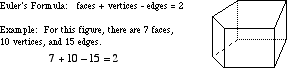# eulers formula (polyhedra)

Euler's Formula (Polyhedra)

The equation below:

(number of faces) + (number of vertices) – (number of edges) = 2

This formula is true for all convexpolyhedra as well as many types of concave polyhedra.

Note: Euler is pronounced "Oiler".